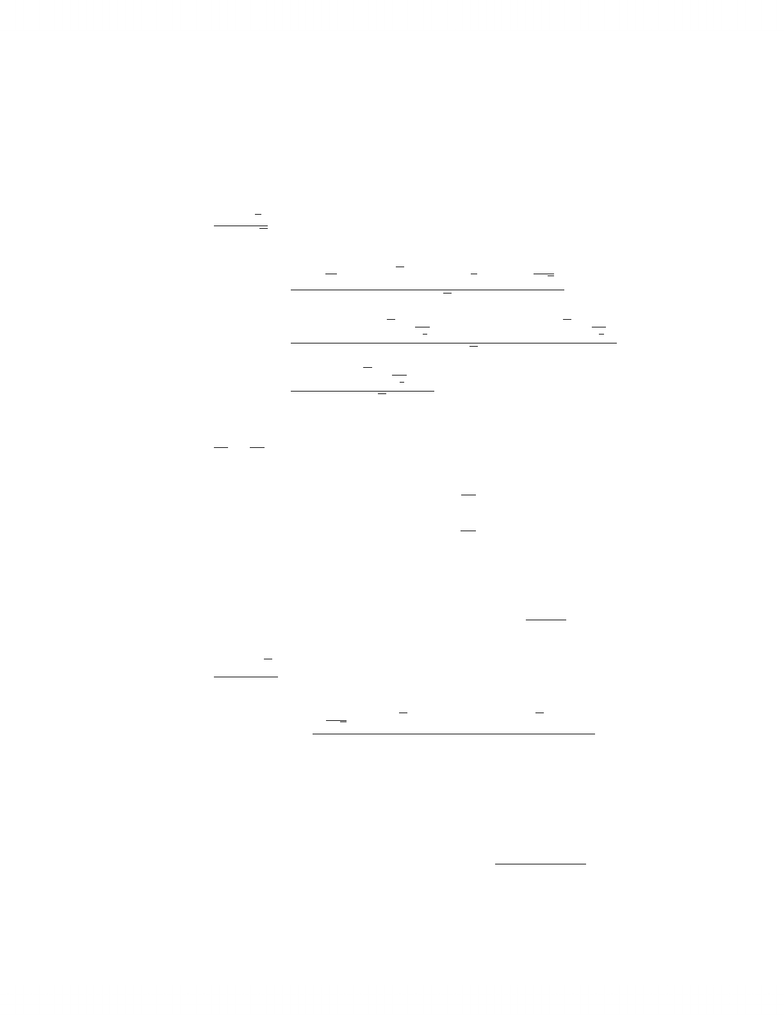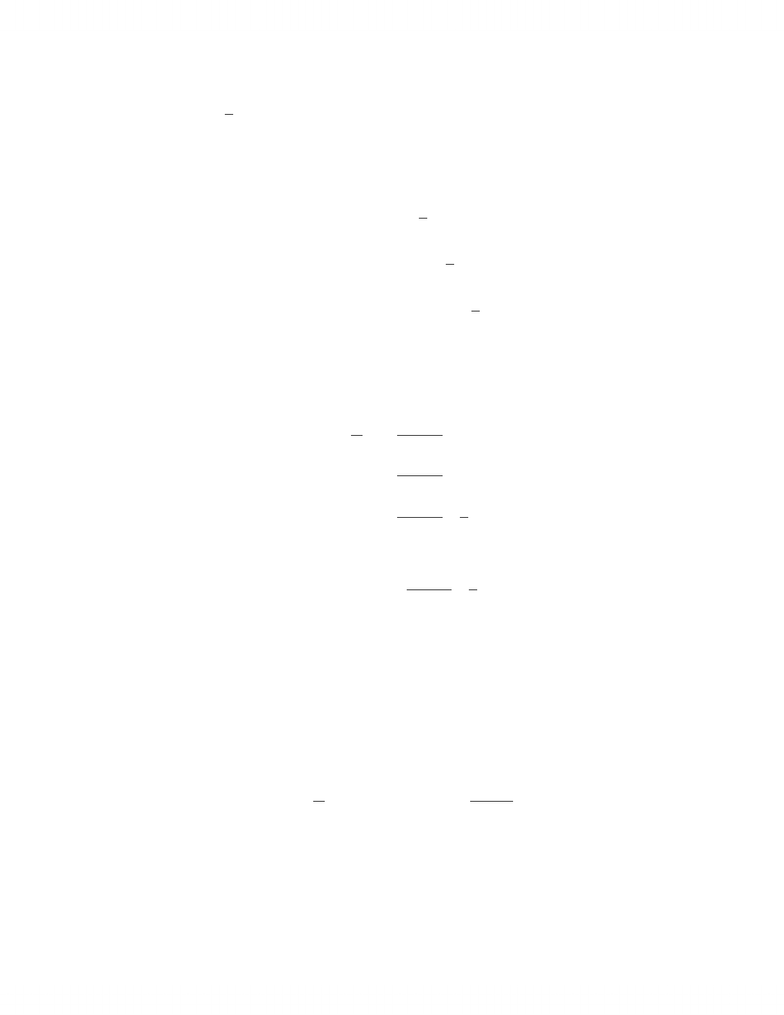Study Guides (390,000)
CA (150,000)
Western (10,000)
MATH (100)
Quiz

# Mathematics 0110A/B Study Guide - Quiz Guide: Quotient Rule

Department
Mathematics
Course Code
MATH 0110A/B
Professor
Chris Brandl
Study Guide
Quiz

This preview shows pages 1-2. to view the full 6 pages of the document.MATH 127 Fall 2012
Assignment 6 Solutions
1. Compute the indicated derivatives for the following functions.
(a) f(x) = 6x2
x
x2+x,f0(x)
f0(x) = 6 + 2
x2)(x2+x)(6x2
x2x+1
2x
(x2+x)2
=6x2+ 2 + 6x+2
x
3
212x24+3x1
x
3
2
(x2+x)2
=6x2+ 3x+3
x
3
2+ 6
(x2+x)2
(b) f(x) = x2
22
x2,f00(x)
f0(x) = x+4
x3
f00(x)=112
x4
(c) f(x) = ex2+1 ln (x2+ 1), f0(x)
f0(x) = (2x)ex2+1 ln (x2+ 1) + ex2+1 2x
x2+ 1
(d) f(x) = cos (πx)
sin (x),f0(x)
f0(x) = π
2xsin (πx)(sin (x)) cos (πx) cos (x)
(sin (x))2
2. Find f0(x)
(a) f(x) = xπarctan(sin (x3))
f0(x) = πxπ1arctan(sin (x3)) + xπ3x2cos (x3)
1 + (sin (x3))2
1

Only pages 1-2 are available for preview. Some parts have been intentionally blurred.(b) f(x) = 1
xln(x)
We will use logarithmic diﬀerentiation to ﬁnd the indicated derivative. We ﬁrst
take the logarithm of both sides of the equation and simplify.
y=1
xln(x)
ln (y) = ln 1
xln(x)!
= ln (x) ln 1
x
=(ln (x))2
Diﬀerentiating both sides we get
y0
y=2 ln (x)
x
y0=2 ln (x)
xy
=2 ln (x)
x1
xln(x)
Thus, we have
f0(x) = 2 ln (x)
x1
xln(x)
(c) f(x) = (cos (x))x
We will compute the derivative of (cos (x))xusing logarithmic diﬀerentiation.
y= (cos (x))x
ln (y) = ln ((cos (x))x)
=xln (cos (x))
y0
y= ln (cos (x)) + xsin (x)
cos (x)
y0= (ln (cos (x)) xtan (x))y
= (ln (cos (x)) xtan (x))(cos (x))x
2